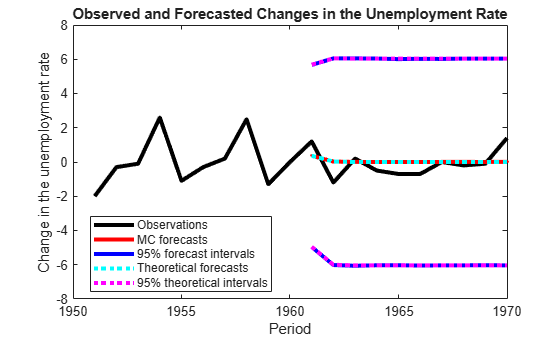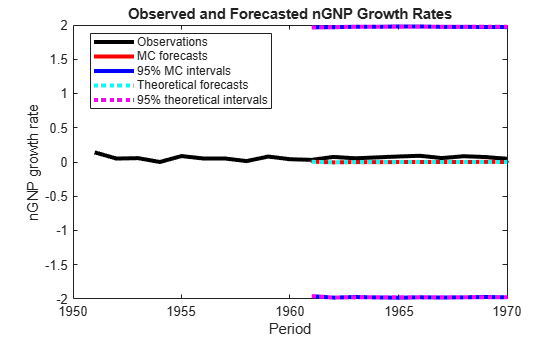# Forecast State-Space Model Using Monte-Carlo Methods

This example shows how to forecast a state-space model using Monte-Carlo methods, and to compare the Monte-Carlo forecasts to the theoretical forecasts.

Suppose that the relationship between the change in the unemployment rate (${x}_{1,t}$) and the nominal gross national product (nGNP) growth rate (${x}_{3,t}$) can be expressed in the following, state-space model form.

`$\left[\begin{array}{c}{x}_{1,t}\\ {x}_{2,t}\\ {x}_{3,t}\\ {x}_{4,t}\end{array}\right]=\left[\begin{array}{cccc}{\varphi }_{1}& {\theta }_{1}& {\gamma }_{1}& 0\\ 0& 0& 0& 0\\ {\gamma }_{2}& 0& {\varphi }_{2}& {\theta }_{2}\\ 0& 0& 0& 0\end{array}\right]\left[\begin{array}{c}{x}_{1,t-1}\\ {x}_{2,t-1}\\ {x}_{3,t-1}\\ {x}_{4,t-1}\end{array}\right]+\left[\begin{array}{cc}1& 0\\ 1& 0\\ 0& 1\\ 0& 1\end{array}\right]\left[\begin{array}{c}{u}_{1,t}\\ {u}_{2,t}\end{array}\right]$`

`$\left[\begin{array}{c}{y}_{1,t}\\ {y}_{2,t}\end{array}\right]=\left[\begin{array}{cccc}1& 0& 0& 0\\ 0& 0& 1& 0\end{array}\right]\left[\begin{array}{c}{x}_{1,t}\\ {x}_{2,t}\\ {x}_{3,t}\\ {x}_{4,t}\end{array}\right]+\left[\begin{array}{cc}{\sigma }_{1}& 0\\ 0& {\sigma }_{2}\end{array}\right]\left[\begin{array}{c}{\epsilon }_{1,t}\\ {\epsilon }_{2,t}\end{array}\right],$`

where:

• ${x}_{1,t}$ is the change in the unemployment rate at time t.

• ${x}_{2,t}$ is a dummy state for the MA(1) effect on ${x}_{1,t}$.

• ${x}_{3,t}$ is the nGNP growth rate at time t.

• ${x}_{4,t}$ is a dummy state for the MA(1) effect on ${x}_{3,t}$.

• ${y}_{1,t}$ is the observed change in the unemployment rate.

• ${y}_{2,t}$ is the observed nGNP growth rate.

• ${u}_{1,t}$ and ${u}_{2,t}$ are Gaussian series of state disturbances having mean 0 and standard deviation 1.

• ${\epsilon }_{1,t}$ is the Gaussian series of observation innovations having mean 0 and standard deviation ${\sigma }_{1}$.

• ${\epsilon }_{2,t}$ is the Gaussian series of observation innovations having mean 0 and standard deviation ${\sigma }_{2}$.

Load the Nelson-Plosser data set, which contains the unemployment rate and nGNP series, among other things.

`load Data_NelsonPlosser`

Preprocess the data by taking the natural logarithm of the nGNP series, and the first difference of each. Also, remove the starting `NaN` values from each series.

```isNaN = any(ismissing(DataTable),2); % Flag periods containing NaNs gnpn = DataTable.GNPN(~isNaN); u = DataTable.UR(~isNaN); T = size(gnpn,1); % Sample size y = zeros(T-1,2); % Preallocate y(:,1) = diff(u); y(:,2) = diff(log(gnpn));```

This example proceeds using series without `NaN` values. However, using the Kalman filter framework, the software can accommodate series containing missing values.

To determine how well the model forecasts observations, remove the last 10 observations for comparison.

```numPeriods = 10; % Forecast horizon isY = y(1:end-numPeriods,:); % In-sample observations oosY = y(end-numPeriods+1:end,:); % Out-of-sample observations```

Specify the coefficient matrices.

```A = [NaN NaN NaN 0; 0 0 0 0; NaN 0 NaN NaN; 0 0 0 0]; B = [1 0; 1 0; 0 1; 0 1]; C = [1 0 0 0; 0 0 1 0]; D = [NaN 0; 0 NaN];```

Specify the state-space model using `ssm`. Verify that the model specification is consistent with the state-space model.

`Mdl = ssm(A,B,C,D)`
```Mdl = State-space model type: ssm State vector length: 4 Observation vector length: 2 State disturbance vector length: 2 Observation innovation vector length: 2 Sample size supported by model: Unlimited Unknown parameters for estimation: 8 State variables: x1, x2,... State disturbances: u1, u2,... Observation series: y1, y2,... Observation innovations: e1, e2,... Unknown parameters: c1, c2,... State equations: x1(t) = (c1)x1(t-1) + (c3)x2(t-1) + (c4)x3(t-1) + u1(t) x2(t) = u1(t) x3(t) = (c2)x1(t-1) + (c5)x3(t-1) + (c6)x4(t-1) + u2(t) x4(t) = u2(t) Observation equations: y1(t) = x1(t) + (c7)e1(t) y2(t) = x3(t) + (c8)e2(t) Initial state distribution: Initial state means are not specified. Initial state covariance matrix is not specified. State types are not specified. ```

Estimate the model parameters, and use a random set of initial parameter values for optimization. Restrict the estimate of ${\sigma }_{1}$ and ${\sigma }_{2}$ to all positive, real numbers using the `'lb'` name-value pair argument. For numerical stability, specify the Hessian when the software computes the parameter covariance matrix, using the `'CovMethod'` name-value pair argument.

```rng(1); params0 = rand(8,1); [EstMdl,estParams] = estimate(Mdl,isY,params0,... 'lb',[-Inf -Inf -Inf -Inf -Inf -Inf 0 0],'CovMethod','hessian');```
```Method: Maximum likelihood (fmincon) Sample size: 51 Logarithmic likelihood: -170.92 Akaike info criterion: 357.84 Bayesian info criterion: 373.295 | Coeff Std Err t Stat Prob ---------------------------------------------------- c(1) | 0.06750 0.16548 0.40791 0.68334 c(2) | -0.01372 0.05887 -0.23302 0.81575 c(3) | 2.71201 0.27039 10.03006 0 c(4) | 0.83815 2.84585 0.29452 0.76836 c(5) | 0.06273 2.83473 0.02213 0.98235 c(6) | 0.05197 2.56875 0.02023 0.98386 c(7) | 0.00273 2.40763 0.00113 0.99910 c(8) | 0.00016 0.13942 0.00113 0.99910 | | Final State Std Dev t Stat Prob x(1) | -0.00000 0.00273 -0.00033 0.99973 x(2) | 0.12237 0.92954 0.13164 0.89527 x(3) | 0.04049 0.00016 256.76330 0 x(4) | 0.01183 0.00016 72.51366 0 ```

`EstMdl` is an `ssm` model, and you can access its properties using dot notation.

Filter the estimated, state-space model, and extract the filtered states and their variances from the final period.

`[~,~,Output] = filter(EstMdl,isY);`

Modify the estimated, state-space model so that the initial state means and covariances are the filtered states and their covariances of the final period. This sets up simulation over the forecast horizon.

```EstMdl1 = EstMdl; EstMdl1.Mean0 = Output(end).FilteredStates; EstMdl1.Cov0 = Output(end).FilteredStatesCov;```

Simulate `5e5` paths of observations from the fitted, state-space model `EstMdl`. Specify to simulate observations for each period.

```numPaths = 5e5; SimY = simulate(EstMdl1,10,'NumPaths',numPaths);```

`SimY` is a `10`-by- `2`-by- `numPaths` array containing the simulated observations. The rows of `SimY` correspond to periods, the columns correspond to an observation in the model, and the pages correspond to paths.

Estimate the forecasted observations and their 95% confidence intervals in the forecast horizon.

```MCFY = mean(SimY,3); CIFY = quantile(SimY,[0.025 0.975],3);```

Estimate the theoretical forecast bands.

```[Y,YMSE] = forecast(EstMdl,10,isY); Lb = Y - sqrt(YMSE)*1.96; Ub = Y + sqrt(YMSE)*1.96;```

Plot the forecasted observations with their true values and the forecast intervals.

```figure h = plot(dates(end-numPeriods-9:end),[isY(end-9:end,1);oosY(:,1)],'-k',... dates(end-numPeriods+1:end),MCFY(end-numPeriods+1:end,1),'.-r',... dates(end-numPeriods+1:end),CIFY(end-numPeriods+1:end,1,1),'-b',... dates(end-numPeriods+1:end),CIFY(end-numPeriods+1:end,1,2),'-b',... dates(end-numPeriods+1:end),Y(:,1),':c',... dates(end-numPeriods+1:end),Lb(:,1),':m',... dates(end-numPeriods+1:end),Ub(:,1),':m',... 'LineWidth',3); xlabel('Period') ylabel('Change in the unemployment rate') legend(h([1,2,4:6]),{'Observations','MC forecasts',... '95% forecast intervals','Theoretical forecasts',... '95% theoretical intervals'},'Location','Best') title('Observed and Forecasted Changes in the Unemployment Rate')``````figure h = plot(dates(end-numPeriods-9:end),[isY(end-9:end,2);oosY(:,2)],'-k',... dates(end-numPeriods+1:end),MCFY(end-numPeriods+1:end,2),'.-r',... dates(end-numPeriods+1:end),CIFY(end-numPeriods+1:end,2,1),'-b',... dates(end-numPeriods+1:end),CIFY(end-numPeriods+1:end,2,2),'-b',... dates(end-numPeriods+1:end),Y(:,2),':c',... dates(end-numPeriods+1:end),Lb(:,2),':m',... dates(end-numPeriods+1:end),Ub(:,2),':m',... 'LineWidth',3); xlabel('Period') ylabel('nGNP growth rate') legend(h([1,2,4:6]),{'Observations','MC forecasts',... '95% MC intervals','Theoretical forecasts','95% theoretical intervals'},... 'Location','Best') title('Observed and Forecasted nGNP Growth Rates')```